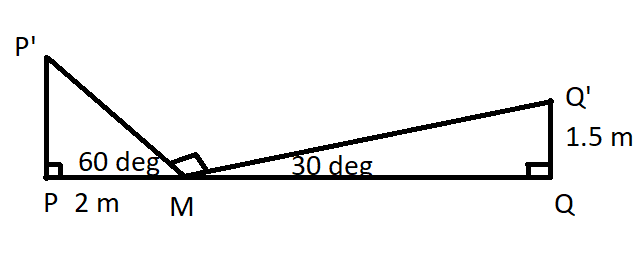Question 16

# A man, from the foot of the building P, walks towards the building Q. After walking for 2 mts, hefinds that buildings P and Q makean angle of elevation of $$60^{0}$$ and $$30^{0}$$ respectively. If building Q is1.5 mts high, find the distance between the tops of both buildings P and Q.

Solution

The situation given in the problem can be summarised as:By 30-60-90 theorem, we can say that P'M = 4 meters and MQ' = 3 meters
Also, triangle P'MQ' would be a right angled triangle.
Hence, P'Q' would be the hypotenuse of length = root(16+9) = 5 meters